Courses

# Properties of Rational Numbers Class 8 Notes | EduRev

## Mathematics (Maths) Class 8

Created by: Indu Gupta

## Class 8 : Properties of Rational Numbers Class 8 Notes | EduRev

The document Properties of Rational Numbers Class 8 Notes | EduRev is a part of the Class 8 Course Mathematics (Maths) Class 8.
All you need of Class 8 at this link: Class 8

## Properties of Rational Numbers

The major properties of rational numbers are:

• Closure Property
• Commutative Property
• Associative Property
• Distributive Property

Closure Property: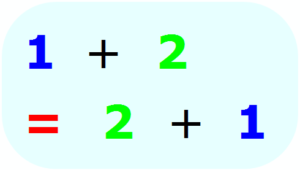1) Addition of Rational Numbers:
The closure property states that for any two rational numbers a and b, a + b is also a rational number.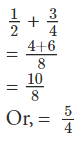The result is a rational number. So we say that rational numbers are closed under addition.
2) Subtraction of Rational Numbers:
The closure property states that for any two rational numbers a and b, a – b is also a rational number.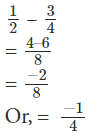The result is a rational number. So the rational numbers are closed under subtraction.
3) Multiplication of Rational Numbers:
The closure property states that for any two rational numbers a and b, a × b is also a rational number.
1/2 x 3/4
= 6/8
The result is a rational number. So rational numbers are closed under multiplication.
4) Division of Rational Numbers:
The closure property states that for any two rational numbers a and b, a ÷ b is also a rational number.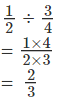The result is a rational number. But we know that any rational number a, a ÷ 0 is not defined. So rational numbers are not closed under division. But if we exclude 0, then all the rational numbers are closed under division.
Commutative Property:
For any two rational numbers a and b, a + b = b+ a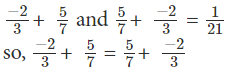We see that the two rational numbers can be added in any order. So addition is commutative for rational numbers.
2. Subtraction:
For any two rational numbers a and b, a – b ≠ b –  a. Given are the two rational numbers 5/3 and  1/4,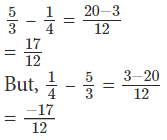So subtraction is not commutative for rational numbers.
3. Multiplication:
For any two rational numbers a and b, a × b = b × a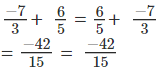We see that the two rational numbers can be multiplied in any order. So multiplication is commutative for rational numbers.
4. Division:
For any two rational numbers a and b, a ÷ b ≠ b ÷ a. Given are the two rational numbers 5/3 and  1/4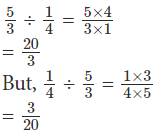We see that the expressions on both the sides are not equal. So division is not commutative for rational numbers.
Associative Property:
Take any three rational numbers a, b and c. Firstly add a and b and then add c to the sum. (a + b) + c. Now again add b and c and then a to the sum, a + (b + c). Is (a + b) + c and a + (b + c) same? Yes and this is how associative property works. It states that you can add or multiply numbers regardless of how they are grouped.

For example, given numbers are 5, -6 and 2/3
( 5 – 6 ) + 2/3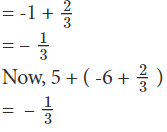In both the groups the sum is the same.

• Addition and multiplication are associative for rational numbers.
• Subtraction and division are not associative for rational numbers.

Distributive Property:
Distributive property states that for any three numbers x, y and z we have

x × ( y + z ) = (x × y) +( x × z)

Solved Examples for You:

Question: …………….. are not associative for rational numbers.
B. Subtraction and multiplication
C. Subtraction and division
Ans: C. When all three rational numbers are subtracted or divided in an order, the result obtained will change if the order is changed. So, subtraction and division are not associative for rational numbers.

Negative of a number:
While studying integers you have come across negatives of integers. What is the negative of 1?
It is – 1 because 1 + (– 1) = (–1) + 1 = 0
So, what will be the negative of (–1)?
It will be 1.

Also, 2 + (–2) = (–2) + 2 = 0, so we say 2 is the negative or additive inverse of –2 and vice-versa. In general, for an integer a, we have, a + (– a) = (– a) + a = 0; so, a is the negative of – a and – a is the negative of a.

For the rational number 2/3 , we have,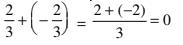Also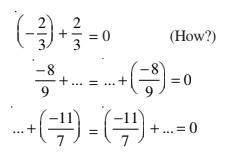In general, for a rational number a/b , we have,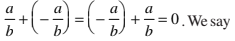that - a/b is the additive inverse of  of a/b and a/b is the additive inverse of (- a/b).

Reciprocal of a Rational  Number:
The reciprocal of a rational number.

For every non-zero rational number a/b there exists a rational number b/a such that
a/b × b/a = 1 = b/a × a/b
The rational number b/a is called the multiplicative inverse or reciprocal of a/b and is denoted by (a/b)-1.
The reciprocal of 12 is 1/12
The reciprocal of 5/16 is 16/5.
The reciprocal of 3/4 is 4/3 i.e., (3/4)-1 = 4/3.
The reciprocal of -5/12 is 12/-5 i.e., (-5/12)-1 = 12/-5.
The reciprocal of (-14)/17 is 17/-14 i.e., (-17)/14.
The reciprocal of -8 is 1/-8 i.e., (-1)/8.
The reciprocal of -5 is 1/-5, since -5 × 1/-5 = -5/1 × 1/-5 = -5 × 1/-5 × 1 = 1.

Note: The reciprocal of 1 is 1 and the reciprocal of -1 is -1. 1 and -1 are the only rational numbers which are their own reciprocals. No other rational number is its own reciprocal.

We know that there is no rational number which when multiplied with 0, gives 1. Therefore, rational number 0 has no reciprocal or multiplicative inverse.

Solved example on reciprocal of a rational number:
Ques 1. Write the reciprocal of each of the following rational numbers:
(i) 5
(ii) -15
(iii) 7/8
(iv) -9/13
(v) 11/-19
Ans:
(i) The reciprocal of 5 is 1/5 i.e., (5)-1 = 1/5.
(ii) The reciprocal of -15 is 1/-15 i.e., (-15)-1 = 1/-15.
(iii) The reciprocal of 7/8 is 8/7 i.e., (7/8)-1 = 8/7.
(iv) The reciprocal of -9/13 is 13/-9 i.e., (-9/13)-1 = 13/-9.
(v) The reciprocal of 11/-19 is -19/11 i.e., (11/-19)-1 = -19/11.

Ques 2. Find the reciprocal of 3/7 × 2/11.
Ans:
3/7 × 2/11
= (3 × 2)/(7 × 11)
= 6/77
Therefore, the reciprocal of 3/7 × 2/11 = Reciprocal of 6/77 = 77/6.

Ques 3. Find the reciprocal of -4/5 × 6/-7.
Ans:
-4/5 × 6/-7
= (-4 × 6)/(5 × -7)
= -24/-35
= 24/35
Therefore, the reciprocal of -4/5 × 6/-7 = Reciprocal of 24/35 = 35/24.

Offer running on EduRev: Apply code STAYHOME200 to get INR 200 off on our premium plan EduRev Infinity!

## Mathematics (Maths) Class 8

212 videos|147 docs|48 tests

,

,

,

,

,

,

,

,

,

,

,

,

,

,

,

,

,

,

,

,

,

;